# statsmodels.tools.eval_measures.aic_sigma¶

statsmodels.tools.eval_measures.aic_sigma(sigma2, nobs, df_modelwc, islog=False)[source]

Akaike information criterion

Parameters: sigma2 : float estimate of the residual variance or determinant of Sigma_hat in the multivariate case. If islog is true, then it is assumed that sigma is already log-ed, for example logdetSigma. nobs : int number of observations df_modelwc : int number of parameters including constant aic : float information criterion

Notes

A constant has been dropped in comparison to the loglikelihood base information criteria. The information criteria should be used to compare only comparable models.

For example, AIC is defined in terms of the loglikelihood as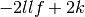in terms of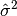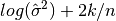in terms of the determinant of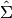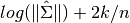Note: In our definition we do not divide by n in the log-likelihood version.

TODO: Latex math

reference for example lecture notes by Herman Bierens

References

http://en.wikipedia.org/wiki/Akaike_information_criterion